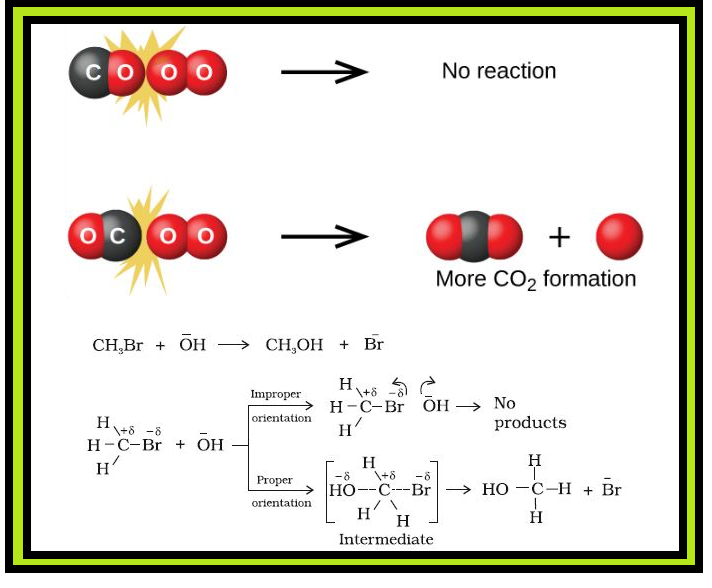Chemistry Collision Theory of Chemical Reactions
Click for Only Video

### Topics Covered :

● Collision Theory

### Collision Theory of Chemical Reactions :=> Though Arrhenius equation is applicable under a wide range of circumstances, collision theory, which was developed by Max Trautz and William Lewis in 1916 -18, provides a greater insight into the energetic and mechanistic aspects of reactions.

color{green}("Postulates of Collision Theory") :

(i) It is based on kinetic theory of gases.

(ii) According to this theory, the reactant molecules are assumed to be hard spheres and reaction is postulated to occur when molecules collide with each other.

color{green}("Collision Frequency") : The number of collisions per second per unit volume of the reaction mixture is known as collision frequency (Z).

(iii) Another factor which affects the rate of chemical reactions is activation energy.

=> For a bimolecular elementary reaction

color{red}(A+B → text(Products))

● Rate of reaction can be expressed as

color{red}(text(Rate) = Z_(AB) e^(-E_a//RT)) ........(4.23)

● color{red}(Z_(AB)) represents the collision frequency of reactants, A and B

● color{red}(e^(-E_a //RT)) represents the fraction of molecules with energies equal to or greater than color{red}(E_a).

● Comparing (4.23) with Arrhenius equation, we can say that A is related to collision frequency.

● Equation (4.23) predicts the value of rate constants fairly accurately for the reactions that involve atomic species or simple molecules but for complex molecules significant deviations are observed.

color{green}("Reason") : This is because all collisions do not lead to the formation of products.

color{green}("Effective Collision") : The collisions in which molecules collide with sufficient kinetic energy (called threshold energy*) and proper orientation, so as to facilitate breaking of bonds between reacting species and formation of new bonds to form products are called as effective collisions.

color{red}("Example") : Formation of methanol from bromoethane depends upon the orientation of reactant molecules as shown in Fig. 4.12. The proper orientation of reactant molecules lead to bond formation whereas improper orientation makes them simply bounce back and no products are formed.

=> To account for effective collisions, another factor P, called the probability or steric factor is introduced. It takes into account the fact that in a collision, molecules must be properly oriented i.e.,

color{red}(text(Rate) = PZ_(AB) e^(-E_a // RT))

color{green}("Conclusion") : Thus, in collision theory activation energy and proper orientation of the molecules together determine the criteria for an effective collision and hence the rate of a chemical reaction.

color{green}("Drawbacks") : (i) It considers atoms/molecules to be hard spheres

(ii) It ignores their structural aspect.